Question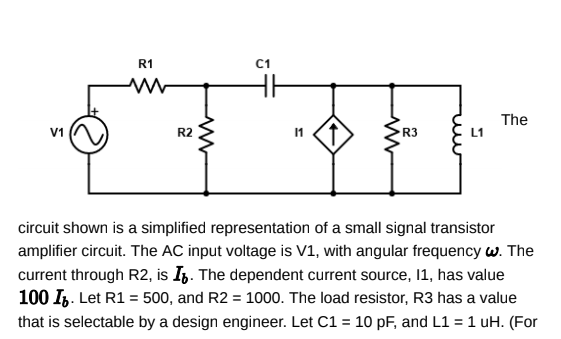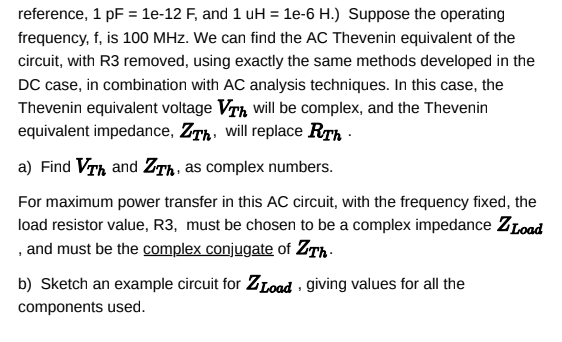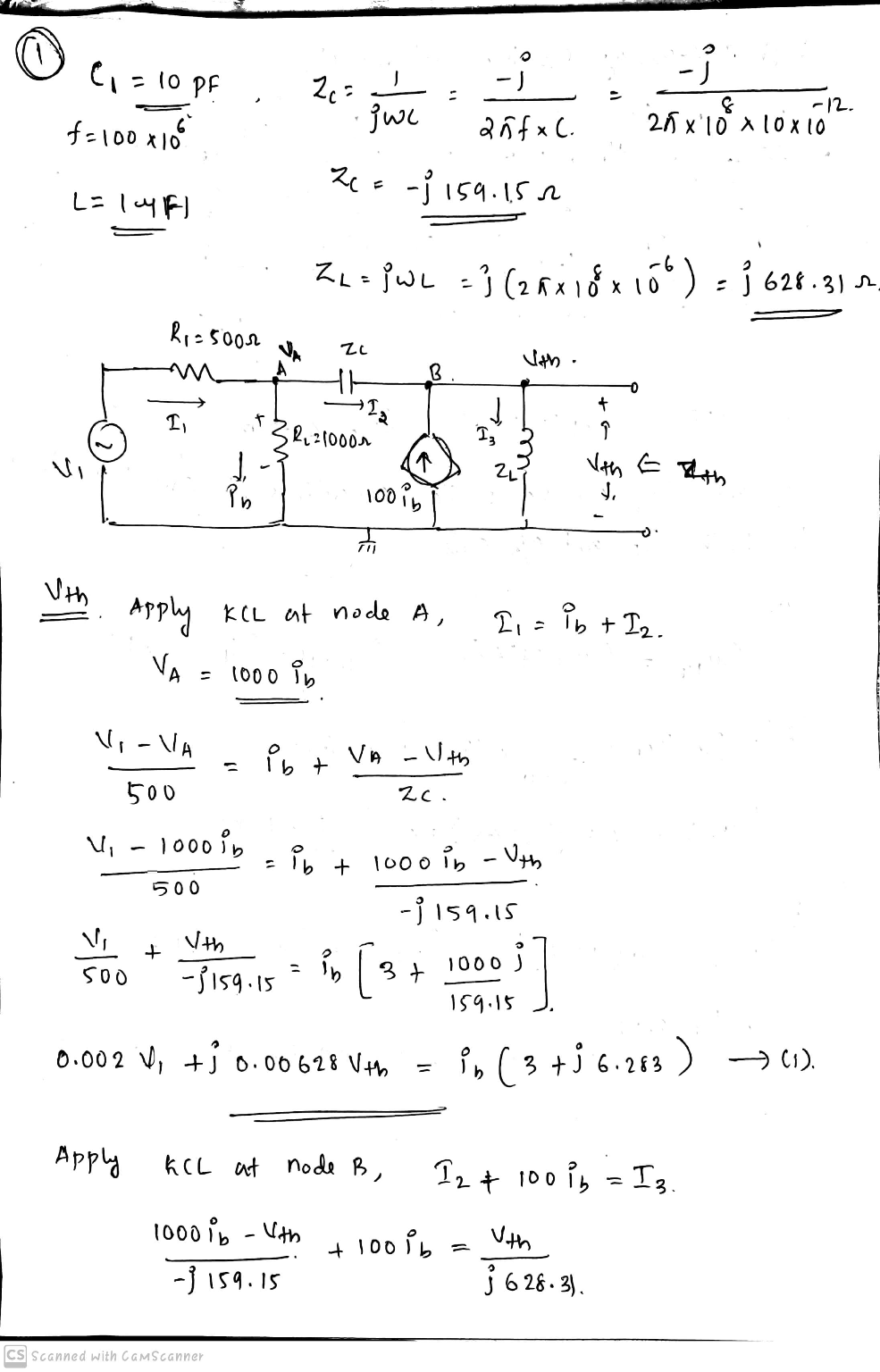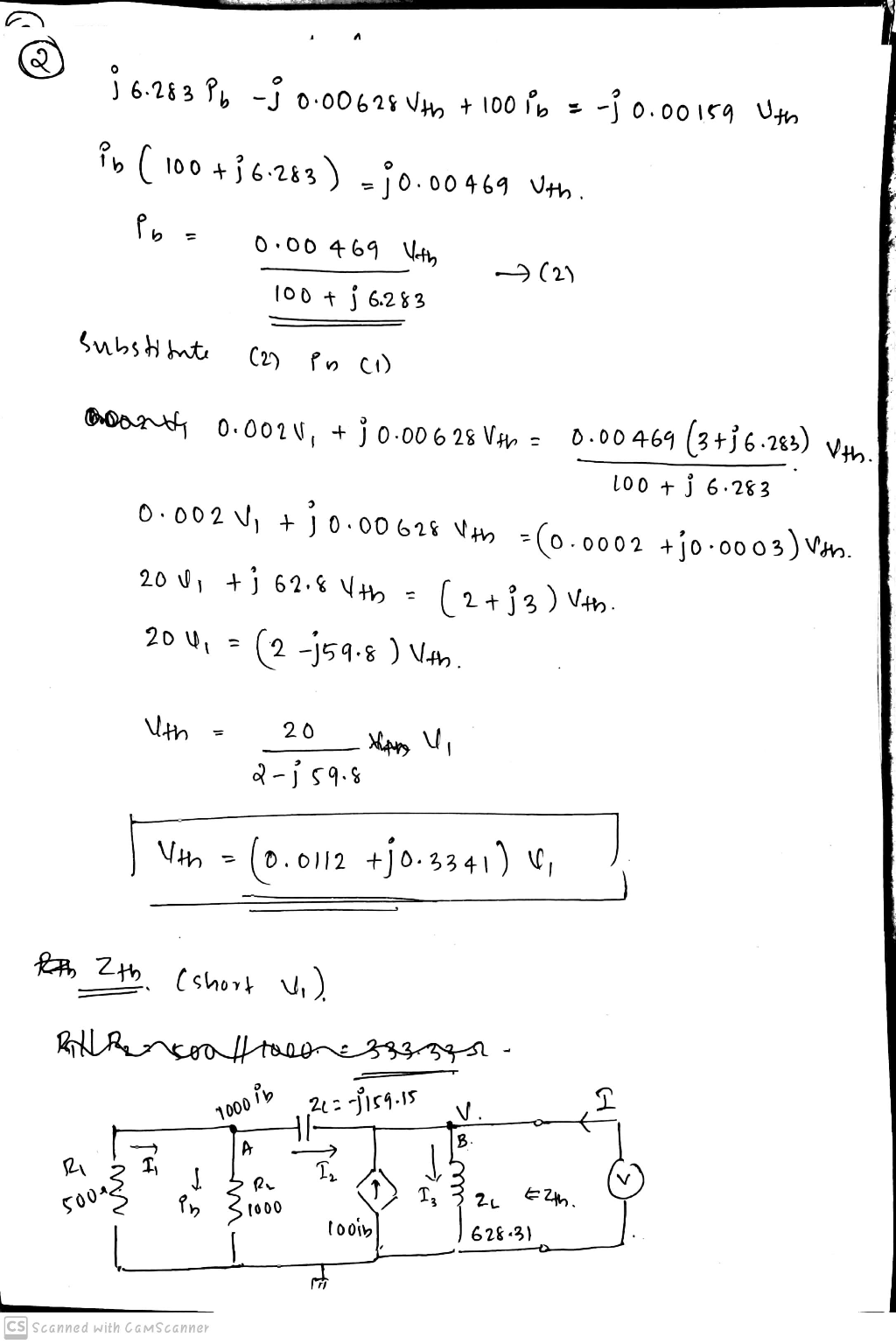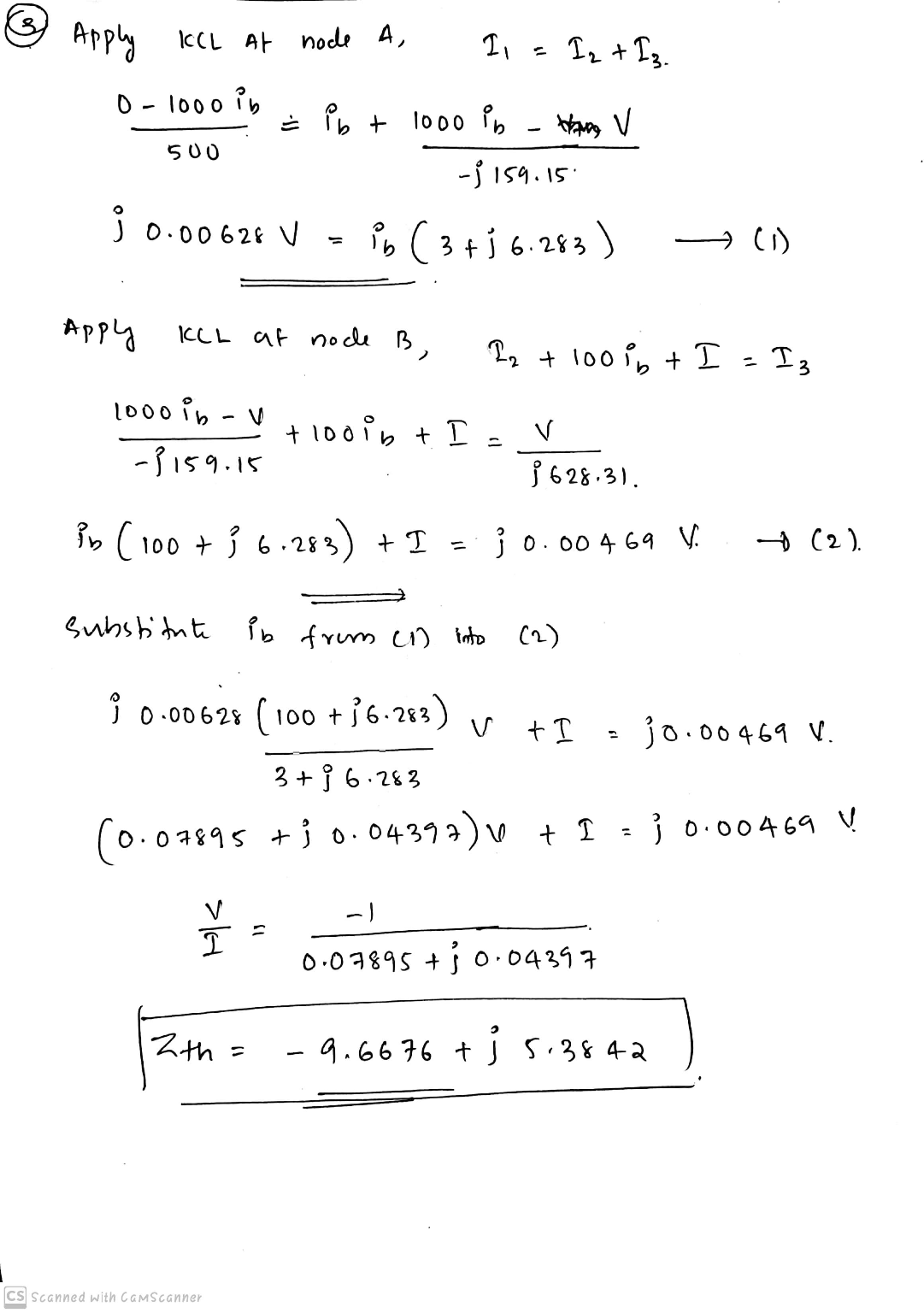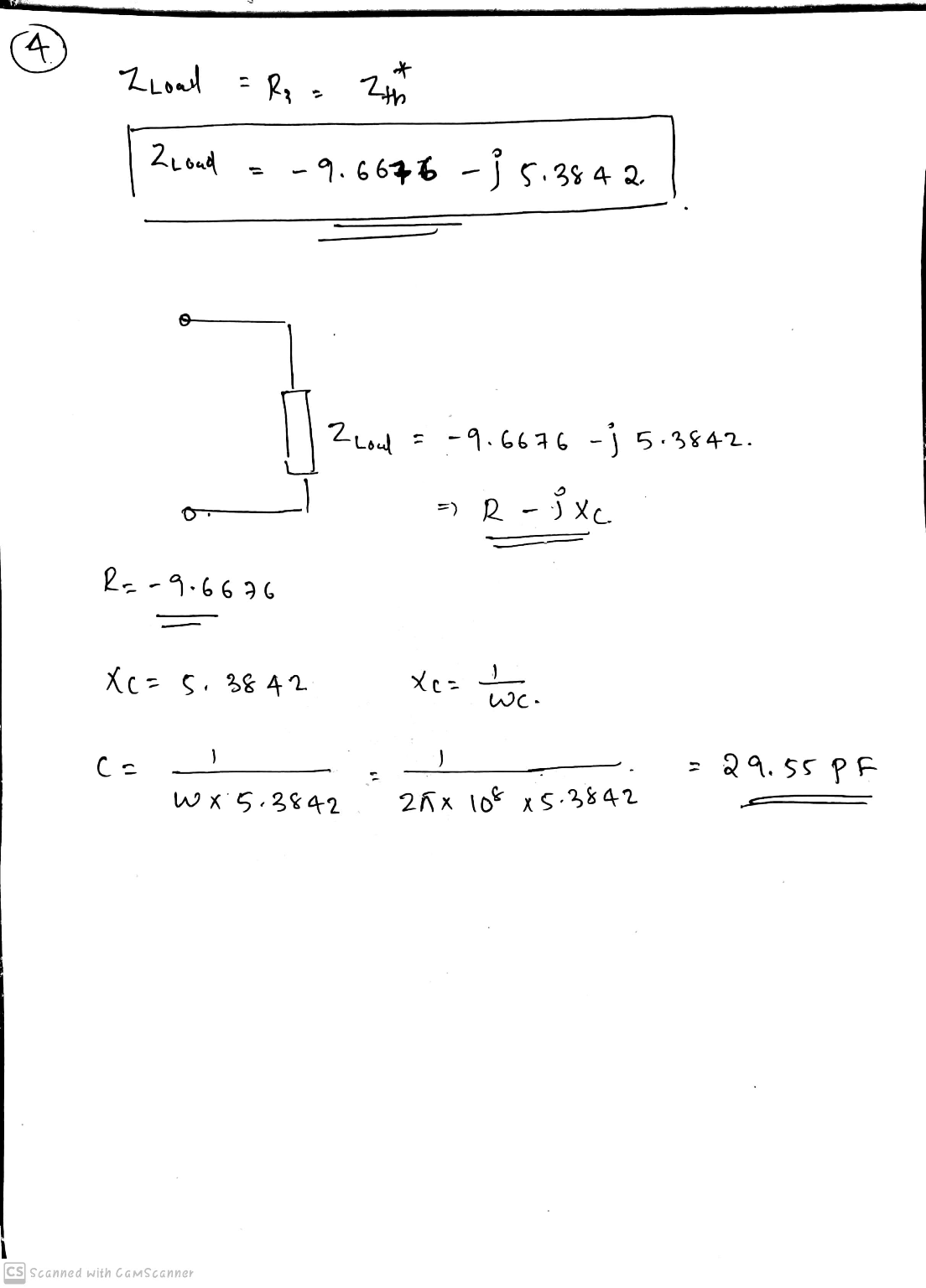#### Earn Coins

Coins can be redeemed for fabulous gifts.

Similar Homework Help Questions
• ### Part B. Wheatstone Bridge Circuit with a Current Source Is R5 R1 R2 Is RL R3 R4 For the circuit a...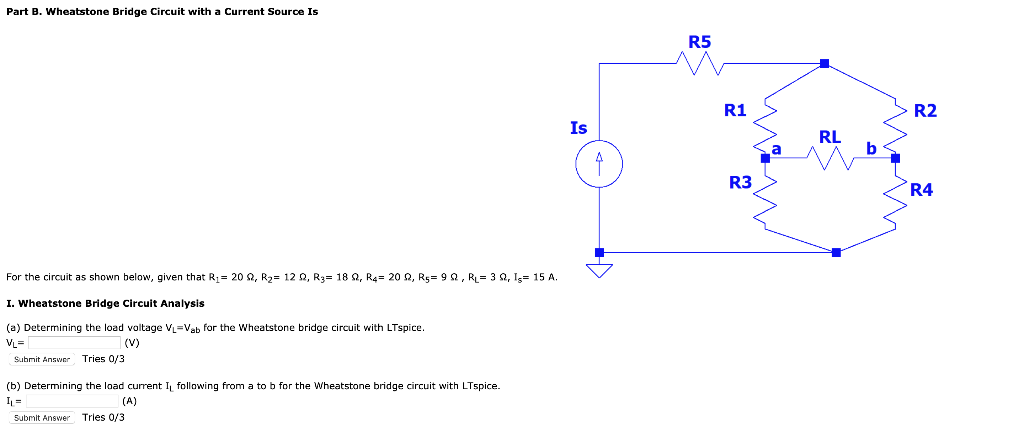Part B. Wheatstone Bridge Circuit with a Current Source Is R5 R1 R2 Is RL R3 R4 For the circuit as shown below, given that R1-20 Ω,R2= 12 Ω, R3-18 Ω, R4= 20 Ω, R5= 9 Ω , R.-3 ΩΊ,-15 A. I. Wheatstone Bridge Circuit Analysis (a) Determining the load voltage VL-Vab for the Wheatstone bridge circuit with LTspice. Subrnit Answer Tries 0/3 (b) Determining the load current I following from a to b for the Wheatstone bridge circuit with...

• ### For the circuit of Figure 1, choose values for resistors R1, R2, and R3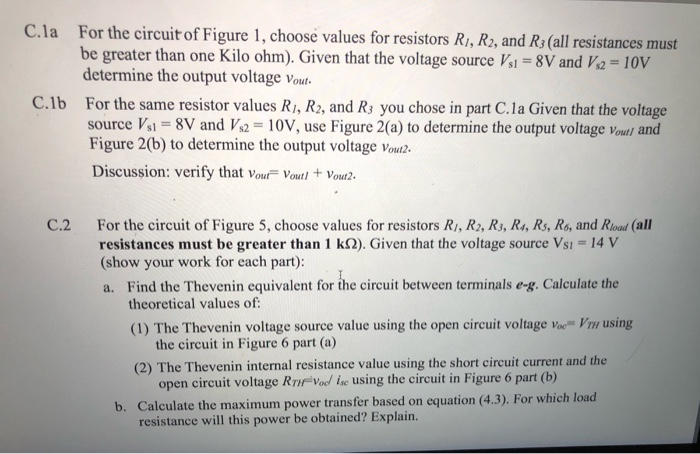C.la For the circuit of Figure 1, choose values for resistors R1, R2, and R3(all resistances must be greater than one Kilo ohm). Given that the voltage source Vs1 = 8V and Vs2 = 10V determine the output voltage Vout. C.1b For the same resistor values Ri, R2, and Rs you chose in part C.la Given that the voltage source Vsi = 8V and Vs2 = 10V, use Figure 2(a) to determine the output voltage Vout/ and Figure 2(b) to determine the output voltage Vout2. Discussion:...

• ### Please show all work Question 3 (3 points) R1 RL R2 R3 The circuit above has...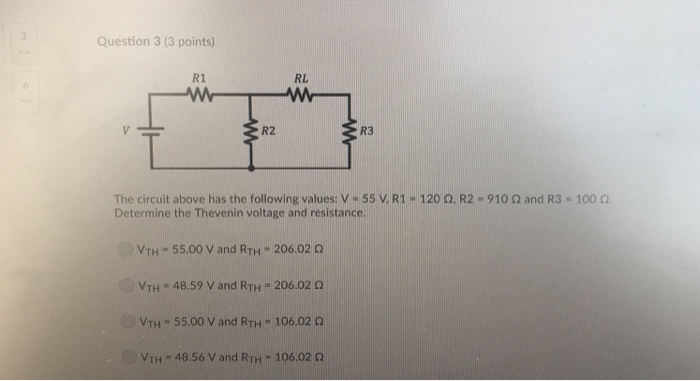Please show all work Question 3 (3 points) R1 RL R2 R3 The circuit above has the following values: V 55 V. R1-120 Ω. R2-910 Ω and R3 = 100 Ω. Determine the Thevenin voltage and resistance (c) VTH-55.00 V and RTH " 206.02 Ω VTH = 48.59 V and RTH = 206.02 Ω VTH 55.00 V and RTH 106.02 0 VTH 48.56 V and RTH 106,022

• ### Question 1 20 pts R3 5 R1 R2 In the circuit 10 15 11 + V1...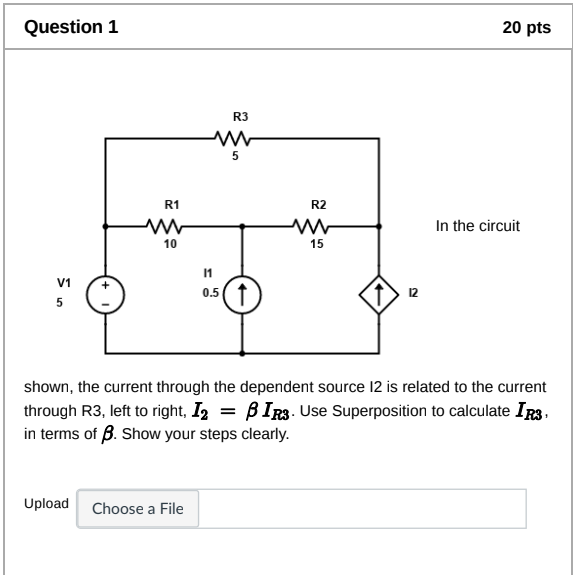Question 1 20 pts R3 5 R1 R2 In the circuit 10 15 11 + V1 5 0.5( 12 shown, the current through the dependent source 12 is related to the current through R3, left to right, I2 = BIR3. Use Superposition to calculate IR3 , in terms of B. Show your steps clearly. Upload Choose a File In this question, use the same circuit as in Question 1, except, make R1 a variable resistor. We are interested in the...

• ### In Figure 3 shown, V1 = 100 millivolts. Further, R1 = 1Kohm, R2 = 10 Kohm,...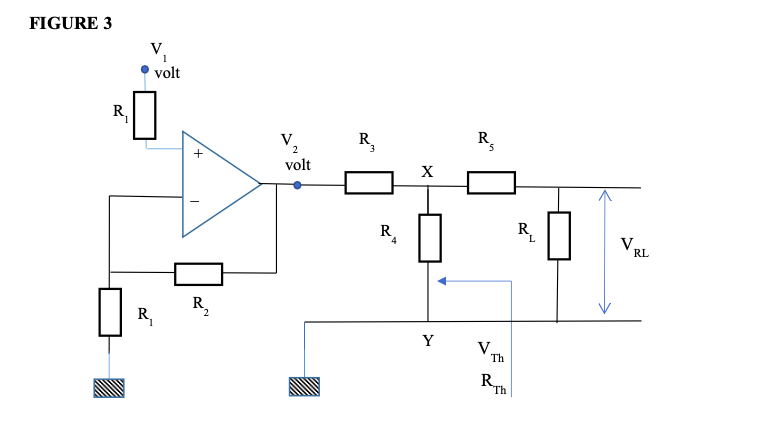In Figure 3 shown, V1 = 100 millivolts. Further, R1 = 1Kohm, R2 = 10 Kohm, R3 = 5 Kohm, R4= 5 Kohm, R5 = 10 Kohm and RL = 1Kohm. (i) Determine the amplified output voltage V2 of the op-amp (of open-loop gain A) in circuit shown in Figure 3. (ii) Suppose this amplified voltage V2 becomes the source voltage to a passive circuit as illustrated in Figure.3. Find Thevenin’s equivalent circuit looking at the terminal XY. Hence, determine...

• ### Find the VTh and the Thevenin equivalent circuit L1 R1 M 100 20:20H V1 50Vpk 0.159Hz...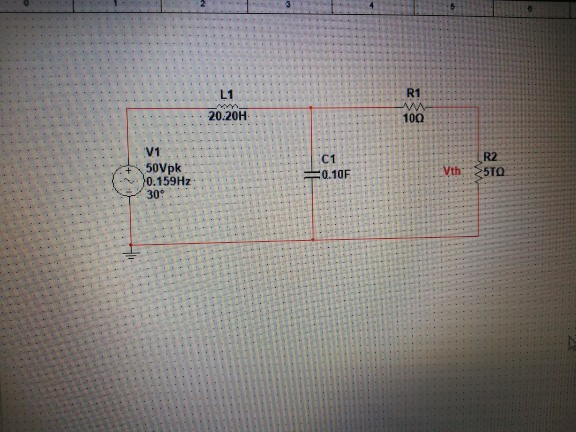Find the VTh and the Thevenin equivalent circuit L1 R1 M 100 20:20H V1 50Vpk 0.159Hz 309 + C1 =0.10F R2 5TQ Vth

• ### Name: ID: 2. For the circuit shown below R1=40, R2=40, and R3=40 circuit shown below in...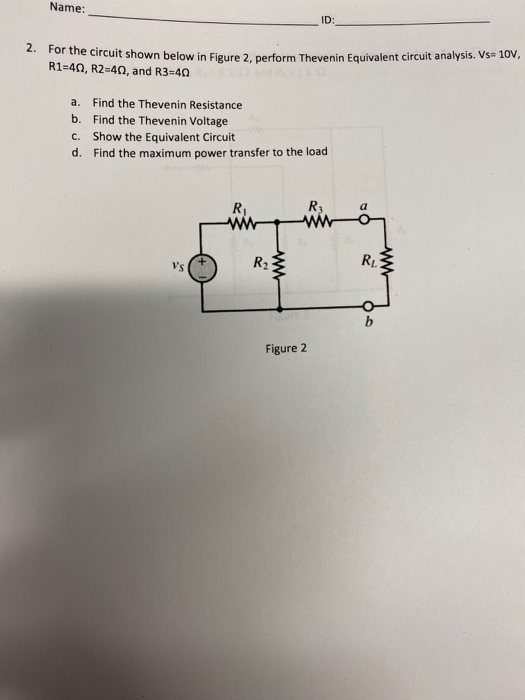Name: ID: 2. For the circuit shown below R1=40, R2=40, and R3=40 circuit shown below in Figure 2. perform Thevenin Equivalent circuit analysis. Vs= 10V, a. Find the Thevenin Resistance b. Find the Thevenin Voltage C. Show the Equivalent Circuit d. Find the maximum power transfer to the load ingina Figure 2

• ### In the circuit shown, R1 = 7 ohm, R2 = 11 ohm, R3 = 9ohm, V1...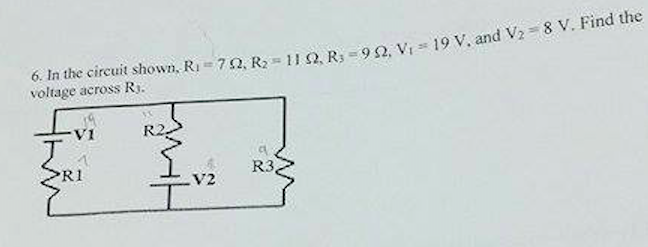In the circuit shown, R1 = 7 ohm, R2 = 11 ohm, R3 = 9ohm, V1 = 19 V, and V2 = 8V. Find the voltage across R3.

• ### For the circuit shown below: In terms of Vo, R1, R2, R3, R4, C1, t, and s what is the time domain...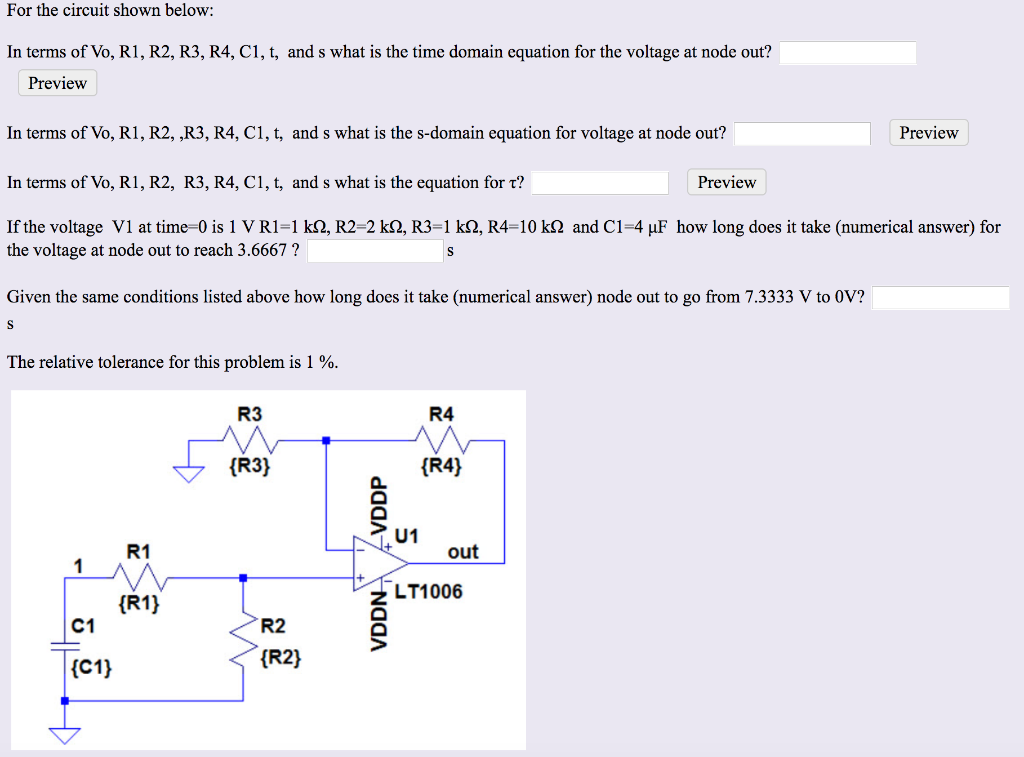For the circuit shown below: In terms of Vo, R1, R2, R3, R4, C1, t, and s what is the time domain equation for the voltage at node out? Preview In terms of Vo, R1, R2, ,R3, R4, C1, t, and s what is the s-domain equation for voltage at node out? In terms of Vo, RI, R2, R3, R4, CI, t, and s what is the equation for τ? If the voltage V1 at time-0 is 1 V R1-1...

• ### Find the Thevenin equivalent with respect to the terminals a, b of the circuit shown using...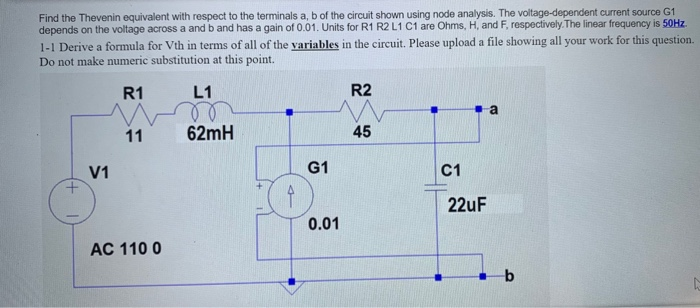Find the Thevenin equivalent with respect to the terminals a, b of the circuit shown using node analysis. The voltage-dependent current source G1 depends on the voltage across a and b and has a gain of 0.01. Units for R1 R2 L1 C1 are Ohms, H and F, respectively. The linear frequency is 50Hz. 1-1 Derive a formula for Vth in terms of all of the variables in the circuit. Please upload a file showing all your work for this...